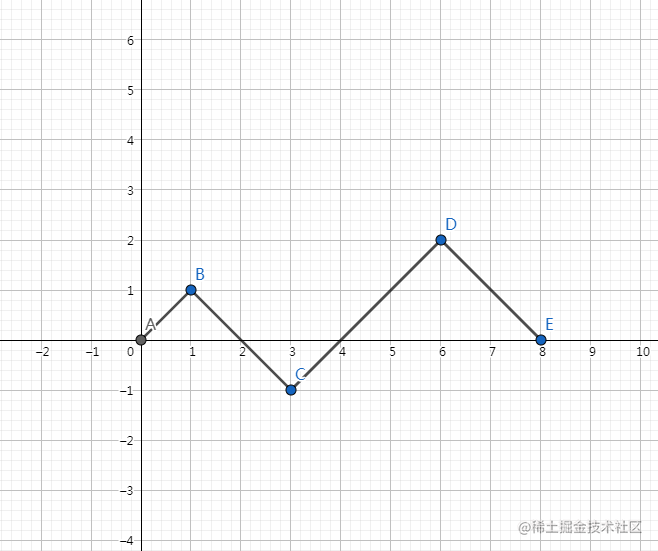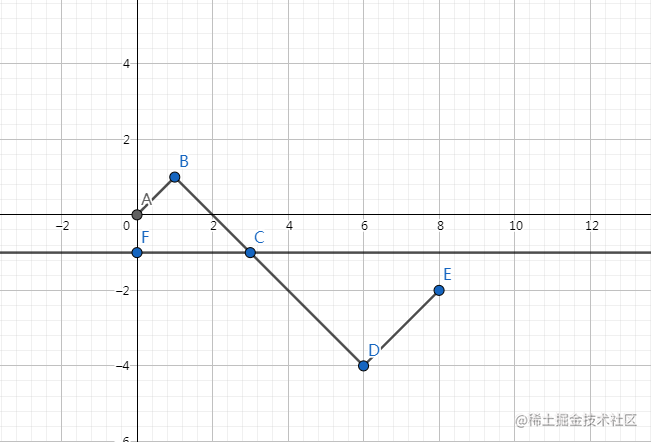LeetCode算法系列 96. 不同的二叉搜索树力扣原题

96. 不同的二叉搜索树

1         3     3      2      1
\       /     /      / \      \
3     2     1      1   3      2
/     /       \                 \
2     1         2                 3

方法一：动态规划

思路与算法1. $G(n)$: 长度为 $n$ 的序列能构成的不同二叉搜索树的个数。
2. $F(i, n)$: 以 $i$ 为根、序列长度为 $n$ 的不同二叉搜索树个数 $(1 \leq i \leq n)$

$G(0) = 1$, $G(1) = 1$

举例说明

$G(n) = \sum_{i=1}^{n}G(i-1) \cdot G(n-i)$

代码实现

class Solution {
public int numTrees(int n) {
int[] G = new int[n + 1];
G = 1;
G = 1;

for (int i = 2; i <= n; ++i) {
for (int j = 1; j <= i; ++j) {
G[i] += G[j - 1] * G[i - j];
}
}
return G[n];
}
}

复杂度分析

• 时间复杂度 : $O(n^2)$，其中 $n$ 表示二叉搜索树的节点个数。$G(n)$ 函数一共有 $n$ 个值需要求解，每次求解需要 $O(n)$ 的时间复杂度，因此总时间复杂度为 $O(n^2)$

• 空间复杂度 : $O(n)$。我们需要 $O(n)$ 的空间存储 $G$ 数组。

方法二：卡特兰数

卡特兰数简介

$f(n)=\frac{C_{2n}^{n}}{n+1}$

$f(n)=\sum_{i=1}^{n}f(i-1)\times f(n-i)$

$f(n)=C_{2n}^{n}-C_{2n}^{n-1}$

卡特兰数推导

1. 我们把 $0$,$1$ 操作扔到一个坐标系中。$1$ 看成向右上方走一步，$0$ 看成向右下角走一步，那么最后构造完后一定走到了$(2n,0)$，如下图：1. 于是我们把与 $y=-1$ 的接触点的右边整条路径以 $y=-1$ 为对称轴翻折，于是终点变为了 $(2n,-2)$，如下图：$C_0 = 1$, $C_{n+1} = \frac{2(2n+1)}{n+2}C_n$

卡特兰数的应用

1. 一个栈(无穷大)的进栈序列为 $1,2,3,..n$ ,有多少个不同的出栈序列?
2. $2n$ 个人排成一行进入剧场。入场费 $5$ 元。其中只有 $n$ 个人有一张 $5$ 元钞票，另外 $n$ 人只有 $10$ 元钞票，剧院无其它钞票，问有多少中方法使得只要有 $10$ 元的人买票，售票处就有 $5$ 元的钞票找零？
3. 一位大城市的律师在她住所以北 $n$ 个街区和以东 $n$ 个街区处工作。每天她走 $2n$ 个街区去上班。如果她从不穿越（但可以碰到）从家到办公室的对角线，那么有多少条可能的道路？
4. 将一个凸多边形区域分成三角形区域的方法数?
5. 在圆上选择 $2n$ 个点,将这些点成对连接起来使得所得到的 $n$ 条线段不相交的方法数?
6. 给定 $n$ 个节点，能构成多少种形状不同的二叉树

代码实现

class Solution {
public int numTrees(int n) {
// 在这里需要用 long 类型防止计算过程中的溢出
long C = 1;
for (int i = 0; i < n; ++i) {
C = C * 2 * (2 * i + 1) / (i + 2);
}
return (int) C;
}
}

复杂度分析

• 时间复杂度 : $O(n)$，其中 $n$ 表示二叉搜索树的节点个数。我们只需要循环遍历一次即可。

• 空间复杂度 : $O(1)$。我们只需要常数空间存放若干变量。